JEE  >  Previous Year Questions: Laws of Motion - 1

# Previous Year Questions: Laws of Motion - 1

Test Description

## 28 Questions MCQ Test Physics Class 11 | Previous Year Questions: Laws of Motion - 1

Previous Year Questions: Laws of Motion - 1 for JEE 2023 is part of Physics Class 11 preparation. The Previous Year Questions: Laws of Motion - 1 questions and answers have been prepared according to the JEE exam syllabus.The Previous Year Questions: Laws of Motion - 1 MCQs are made for JEE 2023 Exam. Find important definitions, questions, notes, meanings, examples, exercises, MCQs and online tests for Previous Year Questions: Laws of Motion - 1 below.
Solutions of Previous Year Questions: Laws of Motion - 1 questions in English are available as part of our Physics Class 11 for JEE & Previous Year Questions: Laws of Motion - 1 solutions in Hindi for Physics Class 11 course. Download more important topics, notes, lectures and mock test series for JEE Exam by signing up for free. Attempt Previous Year Questions: Laws of Motion - 1 | 28 questions in 28 minutes | Mock test for JEE preparation | Free important questions MCQ to study Physics Class 11 for JEE Exam | Download free PDF with solutions
 1 Crore+ students have signed up on EduRev. Have you?
Previous Year Questions: Laws of Motion - 1 - Question 1

### One end of massless rope, which passes over a massless and frictionless pulley P is tied to a hook C while the other end is free. maximum tension that the rope can bear is 360 N. Which what value of maximum safe acceleration (in ms-2) can a man of 60 kg climb down the rope ?         [AIEEE 2002]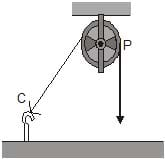Detailed Solution for Previous Year Questions: Laws of Motion - 1 - Question 1

The free body diagram of the person can be drawn as Let the person move down with an acceleration a then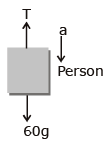60g- T = 60 ⇒ 60g -Tmax = 60 amin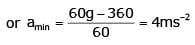Previous Year Questions: Laws of Motion - 1 - Question 2

### Three identical blocks of masses m = 2 kg are drawn by a force F = 10.2N with an acceleration of 0.6 ms-2 on a frictionless surface, then what is the tension (in N) in the string between that blocks B and C ?  [AIEEE 2002]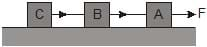Detailed Solution for Previous Year Questions: Laws of Motion - 1 - Question 2

Let the tension between B and C be T

M=2kg

F−T=ma

10.2−T=ma

10.2−T=2×0.6

10.2−T=1.2

10.2−1.2=T

T=9N

Previous Year Questions: Laws of Motion - 1 - Question 3

### A light string passing over a smooth light pulley connects two blocks of masses m1 and m2 (vertically). If the acceleration of the system is g/8,then ratio of the masses is [AIEEE 2002]

Detailed Solution for Previous Year Questions: Laws of Motion - 1 - Question 3

As the string is inextensible, both masses have the same acceleration a. Also, the pulley is massless and frictionless, hence the tension at both ends of the string is the same. Suppose the mass m2 is greater than mass m1, so the heavier mass m2 is accelerating downward and the lighter mass m1 is accelerating upwards.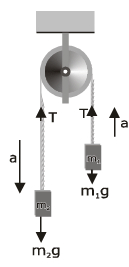Therefore, by Newton's 2nd law

T - m1g =m1a ...(i)

m2g - T = m2a ...(ii)

After solving Eqs. (i) and (ii)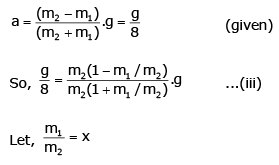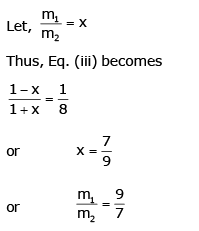So, the ratio of the masses is 9:7.

Previous Year Questions: Laws of Motion - 1 - Question 4

The minimum velocity (in ms-1) with which a car driver must traverse a flat curve of radius 150 m and coefficient of friction 0.6 to avoid skidding is

[AIEEE 2002]

Detailed Solution for Previous Year Questions: Laws of Motion - 1 - Question 4

Using the relation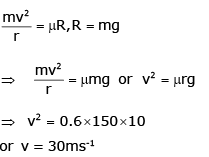Previous Year Questions: Laws of Motion - 1 - Question 5

When forces F1, F2, F3 are acting on a particle of mass m such that F2 and F3are mutually perpendiclar, then the particle remains stationary. If the force F1 is now removed, then the acceleration of the particle is

[AIEEE 2002]

Detailed Solution for Previous Year Questions: Laws of Motion - 1 - Question 5

The particle remains stationary under the acting of three forces F1,F2  and F3 , it means resultant force is zero,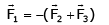Since, in second case F1 is removed (in terms of magnitude we are talking now), the forces acting are F2 and F3 the resultant of which has the mag-nitude as F1, so acceleration of particle is F1 /m in
the direction opposite to that of F1.

Previous Year Questions: Laws of Motion - 1 - Question 6

Two forces are such that the sum of their magnitudes is 18 N and their resultant which has magnitude 12 N, is perpendicular to the smaller force. Then the magnitudes of the forces are

Detailed Solution for Previous Year Questions: Laws of Motion - 1 - Question 6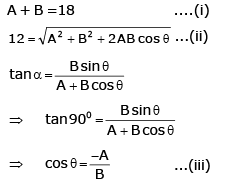Solving Eqs. (i), (ii) and (iii), A = 5N, B = 13N

Previous Year Questions: Laws of Motion - 1 - Question 7

A lift is moving down with acceleration a. A man in the lift drops a ball inside the lift. The acceleration of the ball as observed by the man in the lift and a man standing stationary on the ground are respectively

[AIEEE 2002]

Detailed Solution for Previous Year Questions: Laws of Motion - 1 - Question 7

Apparent weight of ball w' = w -R

R = ma (acting upward)

w' = mg-ma = m(g-a)

Hence, apparent acceleration in the lift is g-a.
Now, if the man is standing stationary on the ground, then the apparent acceleration of the falling ball is g.

Previous Year Questions: Laws of Motion - 1 - Question 8

A rocket witha lift-off mass 3.5 × 104 kg is blasted upwards with an initial acceleration of 10 ms-2. Then the initial thrust of the blast is

[AIEEE 2003]

Detailed Solution for Previous Year Questions: Laws of Motion - 1 - Question 8

Here, thrust force is responsible to accelerate the rocket, so initial thrust of the blast

= ma

= 3.5 × 104 × 10

= 3.5 × 105 N

Previous Year Questions: Laws of Motion - 1 - Question 9

A light spring balance hangs from the hook of the other light spring balance and a block of mass M kg hangs from the former one. Then the true statement about the scale reading is

[AIEEE 2003]

Detailed Solution for Previous Year Questions: Laws of Motion - 1 - Question 9

The arrangement is shown in figure.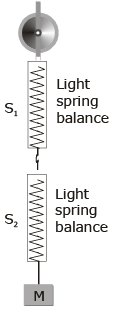Now, draw the free body diagram of the spring balances and block. for equilibrium of block,

T1 = Mg

where T1 = Reading of S2 For equilibrium of S2.
T2 = T1

Where, T2
= Reading of S1 For equilibrium of S1, T2 = T3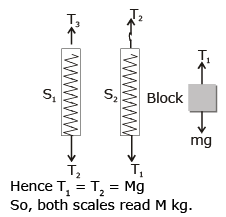Previous Year Questions: Laws of Motion - 1 - Question 10

Consider the following two statements :

A. Linear momentum of a system of particles is zero.

B. kinetic energy of a system of particles is zero.Then

[AIEEE 2003]

Detailed Solution for Previous Year Questions: Laws of Motion - 1 - Question 10

Here B is implying A but A is not implying B, as kinetic energy of a system of particles is zero means speed of each and every Particle is zero. which says that momentum of every particle is zero.
But statement A means linear momentum of a system of particles is zero, which may be true even if particles have equal and opposite momentums and hence, having non-zero kinetic energy.

Previous Year Questions: Laws of Motion - 1 - Question 11

A marble block of mass 2 kg lying on ice when given a velocity of 6 ms-1 is stopped by friction in 10 s. Then the coefficient of friction is

Detailed Solution for Previous Year Questions: Laws of Motion - 1 - Question 11

Let coefficient of friction be μ, then retardation will be μg.
From equation of motion.

v = u +at

0 = 6 - μg × 10

or μ = 6/100 = 0.06

Previous Year Questions: Laws of Motion - 1 - Question 12

A horizontal force of 10 N is necessary to just hold a block stationary against a wall. The coefficient of friction between the block and the wall is 0.2. The weight of the block is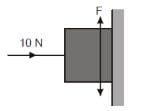[AIEEE 2003]

Detailed Solution for Previous Year Questions: Laws of Motion - 1 - Question 12

Let R be the normal contact force by wall on the block.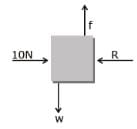R = 10 N
fL = w and f = μR
∴ μR = w
or w = 0.2 x 10 = 2N

Previous Year Questions: Laws of Motion - 1 - Question 13

Three forces start acting simultaneously on a particle moving with velocity . These forces are represented in magnitude and direction by the three sides of a triangle ABC (as shown). The particle will now move with velocity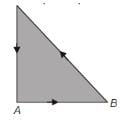[AIEEE 2003]

Detailed Solution for Previous Year Questions: Laws of Motion - 1 - Question 13

Resultant force is zero, as three forces on the particle can be represented in magnitude and direction by three sides of a       triangle in same order. Hence, by Newton's 2nd law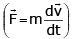Particle velocity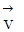will be same

Previous Year Questions: Laws of Motion - 1 - Question 14

A spring balance is attached to the ceiling of a lift. A man hangs his bag on the spring and the spring reads 49 N, when the lift is stationary. If the lift moves downward with an acceleration of 5 ms-2, the reading of the spring balance will be

[AIEEE 2003]

Detailed Solution for Previous Year Questions: Laws of Motion - 1 - Question 14

In stationary position, spring balance reading  => mg = 49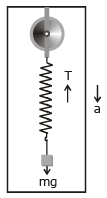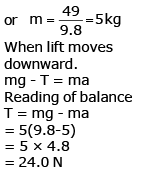Previous Year Questions: Laws of Motion - 1 - Question 15

A block rests on a rough inclined plane making an angle of 30° with the horizontal. The coefficient of static friction between the block and the plane is 0.8. If the frictional force on the block is 10 N, the mass of the block (in kg) is (g = 10 m/s2

[AIEEE 2004]

Detailed Solution for Previous Year Questions: Laws of Motion - 1 - Question 15

Let mass of the block be m.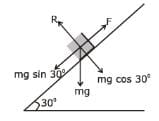Frictional force in rest position F = mg sin 300

[This is static frictional force and may be less than the limiting frictional force]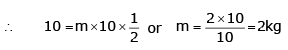Previous Year Questions: Laws of Motion - 1 - Question 16

Two masses m1 = 5 kg and m2 = 4.8 kg tied to a string are hanging over a light frictionless pulley. What is the acceleration of the masses when lift is free to move ? (g = 9.8 ms_2)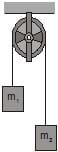[AIEEE 2004]

Detailed Solution for Previous Year Questions: Laws of Motion - 1 - Question 16

On releasing, the motion of the system will be according to figure.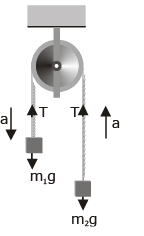m1g - T = m1a    ...(i)

and T-m2g = m2a   ...(ii)

on solving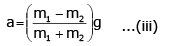Here

m1 = 5 kg , m2 = 4.8 kg

g=9.8 ms-2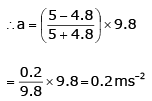Previous Year Questions: Laws of Motion - 1 - Question 17

A machine gun fires a bullet of mass 40 g with a velocity 1200 ms-1. The man holding it, can exert a maximum force of 144 N on the gun. How many bullets can he fire per second at the most ?

[AIEEE 2004]

Detailed Solution for Previous Year Questions: Laws of Motion - 1 - Question 17

The force exerted by machine gun on man's hand in firing a bullet. = change in momentum per second on a bullet or rate of change of momentum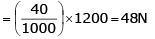The force exerted by man on machine gun = force exerted on man by machine gun = 144 N

Previous Year Questions: Laws of Motion - 1 - Question 18

The upper half of an inclined plane with inclination f is perfectly smooth, while the lower half is rough. A body starting from rest at the top will again come to rest at the bottom, if the coefficient of friction for the lower half is given by

[AIEEE 2005]

Detailed Solution for Previous Year Questions: Laws of Motion - 1 - Question 18

According to work - energy theorem,

w = Δk = 0

⇒ work done by friction + work done by gravity = 0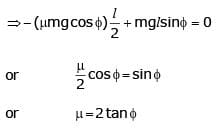Previous Year Questions: Laws of Motion - 1 - Question 19

A smooth block is released at rest on a 45° incline and then slides a distances d. The time taken to slide is n time as much to slide on rough incline than on a smooth incline. The coefficient of friction is

[AIEEE 2005]

Detailed Solution for Previous Year Questions: Laws of Motion - 1 - Question 19

When friction is absent

a1 = gsinθ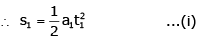When friction is present

a2 = gsin θ - μk gcos θ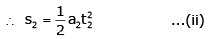From Eqs. (i) and (ii)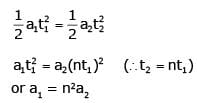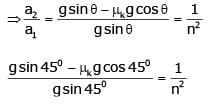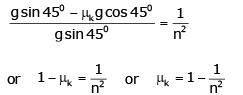Previous Year Questions: Laws of Motion - 1 - Question 20

An angular ring with inner and outer radii R1 and R2 is rolling without slipping with a uniform angular speed. The ratio of the forces experienced by the two particles situated on the inner and outer parts of the ring,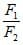is

[AIEEE 2005]

Detailed Solution for Previous Year Questions: Laws of Motion - 1 - Question 20

Since ω is constant, v would also be constant.
So, no net force or torque is acting on ring. The force experienced by any particle is only along radial direction, or we can say the centripetal force.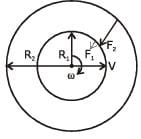The force experienced by inner part, F1 = mω2Rand the force experienced by outer part,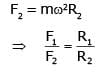Previous Year Questions: Laws of Motion - 1 - Question 21

A particle of mass 0.3 kg is subjected to a force F = - kx with k = 15 Nm-1. What will be its initial acceleration, if it is released from a point 20 cm away from the origin ?

[AIEEE 2005]

Detailed Solution for Previous Year Questions: Laws of Motion - 1 - Question 21

Given,

m= 0.3 kg, x = 20cm and k = 15N/m

F= -kx    ..(i)
and F = ma    ..(ii)
∴   ma = -kx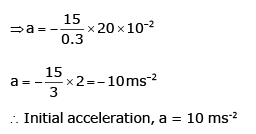Previous Year Questions: Laws of Motion - 1 - Question 22

Consider a car moving on a straight road with a speed of 100 ms-1. The distance at which car can be stopped, is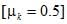[AIEEE 2005]

Detailed Solution for Previous Year Questions: Laws of Motion - 1 - Question 22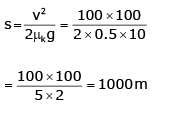Previous Year Questions: Laws of Motion - 1 - Question 23

A block is kept on a frictionless inclined surface with angle of inclination a. The incline is given an acceleration a to keep the block stationary. Then a is equal to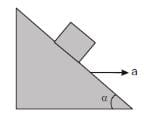[AIEEE 2005]

Detailed Solution for Previous Year Questions: Laws of Motion - 1 - Question 23

In the frame of wedge, the force diagram of block is shown in figure. From free body diagram of wedge.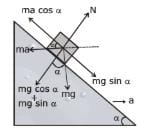For block to remain stationary

ma cos α = mg sin α

or         a = g tan α

Previous Year Questions: Laws of Motion - 1 - Question 24

A player caught a cricket ball of mass 150 g moving at a rate of 20 m/s. If the catching process is completed in 0.1 s, the force of the blow exerted by the ball on the hand of the player is equal to

[AIEEE 2006]

Detailed Solution for Previous Year Questions: Laws of Motion - 1 - Question 24

This is the question based on impulse momentum theorem.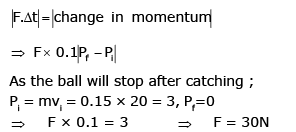Previous Year Questions: Laws of Motion - 1 - Question 25

A ball of mass 0.2 kg is thrown vertically upwards by applying a force by hand. If the hand moves 0.2 m while applying the force and the ball goes upto 2 m height further, find the magnitude of the force. Consider g = 10 m/s2.

[AIEEE 2006]

Detailed Solution for Previous Year Questions: Laws of Motion - 1 - Question 25

The situation is shown in figure. At initial time, the ball is at P, then under the action of a force (exerted by hand) from P to A and then from A to B let acceleration of ball during PA is a ms-2 [assumed to be constant] in upward direction and velocity of ball at A is v m/s.
Then for PA, v2 = 02 +2a × 0.2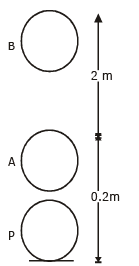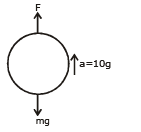For AB, 0 = v2 - 2×g×2 => v2 = 2g × 2

From above equations,

a = 10g =100ms-2

Then for PA, FBD of ball is  F - mg = ma

[F is the force exted by hand on ball] => F = m(g+a) = 0.2(11g)

= 22 N

Previous Year Questions: Laws of Motion - 1 - Question 26

Two fixed frictionless inclined plane making an angle 30° and 60° with the vertical are shown in the figure. Two block A and B are placed on the two planes. What is the relative vertical acceleration of A with respect to B ?

[AIEEE 2010]

Detailed Solution for Previous Year Questions: Laws of Motion - 1 - Question 26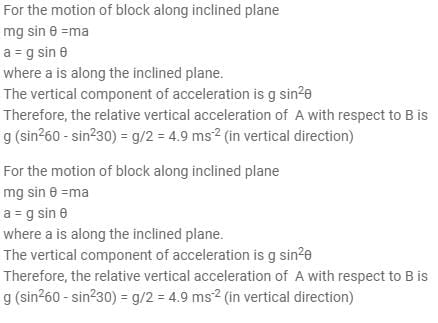Previous Year Questions: Laws of Motion - 1 - Question 27

The figure shows the position-time (x-t) graph of one-dimensional motion of a body of mass 0.4 kg. The magnitude of each impulse is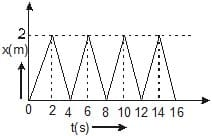[AIEEE 2010]

Detailed Solution for Previous Year Questions: Laws of Motion - 1 - Question 27

From this graph we can see that there is a straight line, so, uniform motion initial velocity = 2/2 = 1 and final velocity = -2/2 = -1 and we know that impulse = change in momentum = Pf - Pi = -0.4 - 0.4 = 0.8

Previous Year Questions: Laws of Motion - 1 - Question 28

The minimum force required to start pushing a body up a rough (frictional coefficient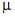) inclined plane is F1 while the minimum force needed to prevent it from sliding down is F2. If the inclined plane makes an angle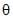from the horizontal such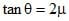, then the ratiois

[AIEEE 2011]

Detailed Solution for Previous Year Questions: Laws of Motion - 1 - Question 28

The minimum force required to start pushing a body up a rough inclined plane is
F1=mgsinθ+ μgcosθ            ......(i)
Minimum force needed to prevent the body from sliding down the inclined plane is
F2=mgsinθ− μgcosθ           ...........(ii)
Divide (i) by (ii), we get
F1/F2
=sinθ+μcosθ/ sinθ−μcosθ
= tanθ+μ/tanθ−μ
= 2μ+μ/2μ−μ
=3              (tanθ=2μ (given))

## Physics Class 11

127 videos|464 docs|210 tests
 Use Code STAYHOME200 and get INR 200 additional OFF Use Coupon Code
Information about Previous Year Questions: Laws of Motion - 1 Page
In this test you can find the Exam questions for Previous Year Questions: Laws of Motion - 1 solved & explained in the simplest way possible. Besides giving Questions and answers for Previous Year Questions: Laws of Motion - 1, EduRev gives you an ample number of Online tests for practice

## Physics Class 11

127 videos|464 docs|210 tests